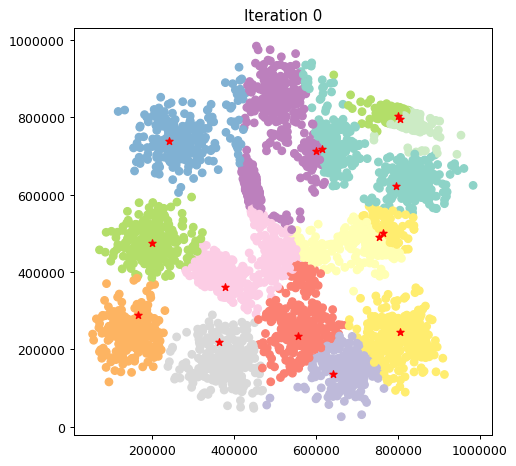## 2D Gaussian clusters¶

### Exercise 1.1¶

To load the dataset, the numpy.loadtxt method can be used. This time, the dataset file contains an header in the first line. We will skip it using the method iself.

In :
import numpy as np
import matplotlib.pyplot as plt
%matplotlib inline

In :
X_gauss = np.loadtxt("2D_gauss_clusters.txt", delimiter=",", skiprows=1)
X_gauss

Out:
array([[845753., 636607.],
[812954., 643720.],
[868217., 609046.],
...,
[706472., 171207.],
[659128., 142611.],
[665898.,  98088.]])

### Exercise 1.2¶

Let's use now matplotlib to explore our 2D data. Since we are likely going to need a scatter plot multiple times, we can define a simple function to handle it.

In :
def plot_2d_scatter(X):
"""Display a 2D scatter plot

:param X: input data points, array
:return: fig, ax, objects
"""
fig, ax = plt.subplots(figsize=(6, 6), dpi=90)
ax.scatter(X[:,0], X[:,1])
return fig, ax # use them for further modifications

In :
_, _ = plot_2d_scatter(X_gauss) # the two underscores let you discard the returned values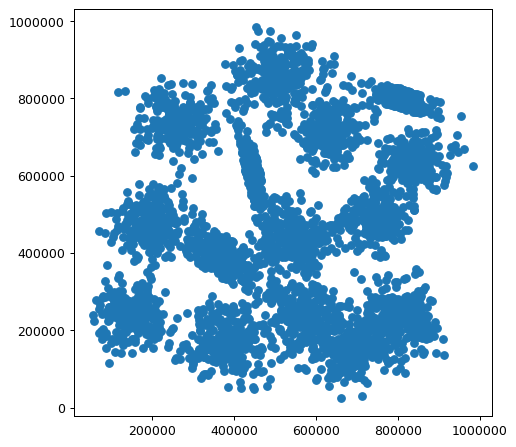Gaussian, globular clusters are easily visible in the euclidean space. At first sight, you can count up to 15 clusters. Most of them have a regular shape, while some others are more elongated. Given this distribution, we known from theory that the K-means algorithm can achieve positive clustering performance (provided that the right number of cluster is chosen).

### Exercises 1.3 and 1.4¶

Let's implement now our version of the K-means algorithm. We will use a custom Python class. The basic blocks will be:

• a fit_predict method exposed to the users of the class
• a stateful structure: we do want to save the final clusters labels and centroids
• some internal plotting functions
• a dump_to_file method to save cluster labels to CSV file (see Exercise 1.4)
In :
class KMeans:

def __init__(self, n_clusters, max_iter=100):
self.n_clusters = n_clusters
self.max_iter = max_iter
self.centroids = None
self.labels = None

def __plot_clusters(self, X, labels, c=None):
fig, ax = plt.subplots(figsize=(6,6), dpi=90)
ax.scatter(X[:,0], X[:,1], c=labels, cmap='Set3')
if c is not None:
ax.scatter(c[:,0], c[:,1], marker="*", color="red")

return fig, ax

def __init_random_centroids(self, X):
c_idx = np.random.randint(0, X.shape, self.n_clusters)
c = X[c_idx] # fancy-indexing
return c

def fit_predict(self, X, plot_clusters=False, plot_steps=5):
"""Run the K-means clustering on X.

:param X: input data points, array, shape = (N,C).
:return: labels : array, shape = N.
"""
# For each point, store the positional index of the closest centroid
nearest = np.empty(X.shape)

c_out = self.__init_random_centroids(X)
for j in range(self.max_iter):
nearest_prev = nearest

# For each point in X, compute the squared distance from each centroid
# dist will have shape: (n_clusters, X.shape)
dist = np.array([np.sum((X-c_out[i])**2, axis=1) for i in range(self.n_clusters)])

# Find the nearest centroid for each point using argmin
# nearest will have shape: (X.shape,)
nearest = dist.argmin(axis=0)

if plot_clusters and (j % plot_steps == 0):
fig, ax = self.__plot_clusters(X, nearest, c_out)
ax.set_title(f"Iteration {j}")

# Early stopping if centroids have not changed
if np.array_equal(nearest, nearest_prev):
print(f"Early stopping at iteration {j}!")
break

# For each cluster, compute the average coordinates considering only points currently
# assigned to it. Then, use them as the new centroid coordinates.
for i in range(self.n_clusters):
c_temp = X[nearest == i].mean(axis=0) # indexing with a mask
if not np.isnan(c_temp).any(): # handle the case of an empty cluster
c_out[i] = c_temp

self.centroids = c_out
self.labels = nearest
return self.labels

def dump_to_file(self, filename):
"""Dump the evaluated labels to a CSV file."""
with open(filename, 'w') as fp:
fp.write('Id,ClusterId\n')
for i, label in enumerate(self.labels):
fp.write(f'{i},{label:d}\n')


There are several things worth noting in the code above:

1. since data are not shuffled during the execution, the class makes use of positional indices. The nearest array is a good example of that. For each point, it keeps track of the index of the centroid of the cluster that point is assigned to. In other words, if the ith item of nearest has value j, we know that the ith point of the dataset is assigned to the jth cluster. Using this convention, the choice of the cluster Ids is straightforward: the ith cluster will have ID = i
2. this is a typical case in which NumPy can significantly speed up the execution. NumPy's broadcasting feature is used to compute the squared distances from each cluster centroid. Then, argmin is used to soft-sort them and find the closest centroid Id. Finally, array_equal lets you efficiently compare the current and previous centroid positions (if we wanted to compare them with a tolerance, we could have used the numpy.allclose method)
3. some internal methods have a double underscore as name prefix. As you should know, Python does not provide the concept of private attributes or methods by choice. However, programmers that define members intended to be private can make use of name mangling (it remains a design choice, it does not prevent other to access to those members). Name mangling is commonplace in many languages. In Python, it is enabled by adding two leading underscore to your member name (actually, you are also restricted to have at most one trailing underscore). You can read more on name mangling here.

We strongly suggest you to stop and try to fully understand the first two points. For your convenience, we commented the shape of the numpy arrays involved at their respective lines.

Let's try now to use our class and save our labels to file.

In :
np.random.seed(0)
kmeans_model = KMeans(10)
l_gauss = kmeans_model.fit_predict(X_gauss)
l_gauss

Early stopping at iteration 11!

/Users/giuseppe/miniconda3/envs/atsa-py37/lib/python3.7/site-packages/numpy/core/numeric.py:2591: RuntimeWarning: invalid value encountered in equal
return bool(asarray(a1 == a2).all())

Out:
array([1, 1, 1, ..., 5, 5, 5])

The algorithm has stopped after 11 iterations since every centroid has not moved between iteration 10 and 11. In other words, at the 11th iteration, no point has changed its closest centroid (i.e. its membership cluster).

Note also line 1 of the previous cell: it initializes the NumPy's random generator with a specific seed. With a fixed random generator, the same points will be chosen as initial centroids among different runs. This is commonplace whenever you want your results to be reproducible. Many scikit-learn classes offer you this functionality via the random_state parameter.

In :
kmeans_model.dump_to_file('gauss_labels.csv')


### Exercise 1.5¶

Let's analyze now the Chameleon dataset just as before.

In :
X_cham = np.loadtxt("chameleon_clusters.txt", delimiter=",", skiprows=1)
X_cham

Out:
array([[ 68.601997, 102.491997],
[454.665985, 264.80899 ],
[101.283997, 169.285995],
...,
[267.605011, 141.725006],
[238.358002, 252.729996],
[159.242004, 177.431   ]])
In :
_, _ = plot_2d_scatter(X_cham)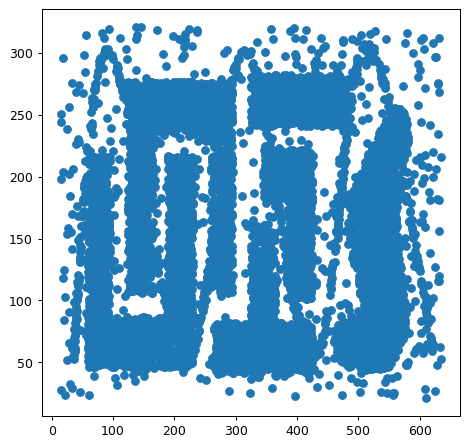As you can notice, there are six interleaved areas with an high density of points and irregular shape. Also, the dataset looks much more noisier (note the sine wave superimposed). Given this distribution, we known from theory that the K-Means algorithm might suffer even choosing the right number of clusters.

### Exercise 1.6¶

It is time to enhance our plotting toolkit. We hence define a new function that highlights the final centroids. Specifically, we can use a different marker style and color for them.

In :
def plot_centroids(X, c, title=None):
fig, ax = plot_2d_scatter(X)
ax.scatter(c[:,0], c[:,1], marker="*", color="red")

if title:
ax.set_title(title)
return fig, ax


Let's now try to inspect the final centroids obtained in both our datasets.

In :
np.random.seed(0)
k_gauss = KMeans(10)
k_cham = KMeans(10)

k_gauss.fit_predict(X_gauss)
k_cham.fit_predict(X_cham)

plot_centroids(X_gauss, k_gauss.centroids, title="Gaussian, K=10")
plot_centroids(X_cham, k_cham.centroids, title="Chameleon, K=10")

Early stopping at iteration 11!
Early stopping at iteration 29!

Out:
(<Figure size 540x540 with 1 Axes>,
<matplotlib.axes._subplots.AxesSubplot at 0x109b78358>)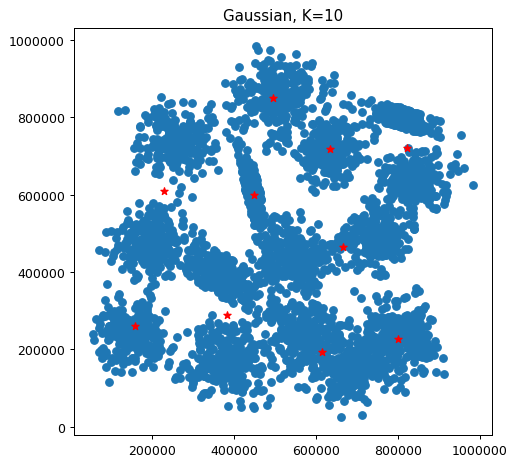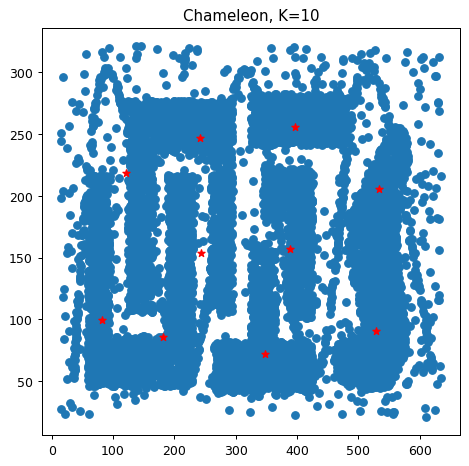We can visually see that the cluster identification might be improved choosing a different K. Our initial choice is too low for Gaussian data while too high for Chameleon.

In :
np.random.seed(0)
k_gauss = KMeans(15)
k_cham = KMeans(6)

k_gauss.fit_predict(X_gauss)
k_cham.fit_predict(X_cham)

plot_centroids(X_gauss, k_gauss.centroids, title="Gaussian, K=15")
plot_centroids(X_cham, k_cham.centroids, title="Chameleon, K=6")

Early stopping at iteration 40!
Early stopping at iteration 24!

Out:
(<Figure size 540x540 with 1 Axes>,
<matplotlib.axes._subplots.AxesSubplot at 0x109a24f98>)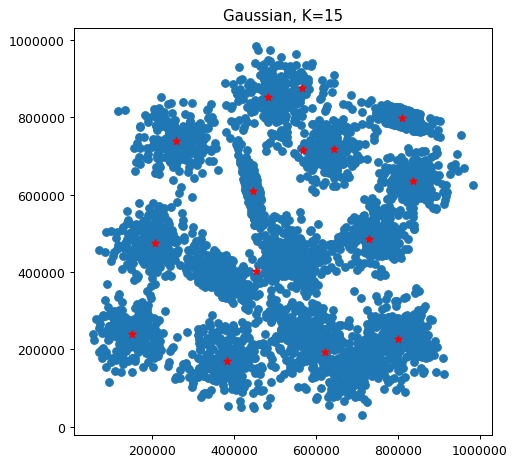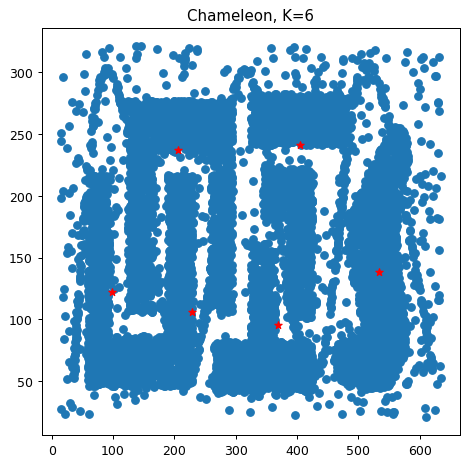Here some interesting happened. On one hand, the Gaussian dataset was clustered in a better way (again, visually speaking). Yet, there are two pairs of centroids in which the two points are close enough to stay in the same globular cluster. This is likely due to the random initialization of centroids (in literature, there exist other approaches rather than randomly picking them). On the other hand, centroids identified in the Chameleon dataset did not match the real data distribution. This is exactly what we expected: the initial distribution has shape and sizes the K-means algorithm can hardly fit.

For the sake of completeness, you can run multiple executions with different seed generators. Results are likely to remain the same for Chameleon clusters. Instead, a different random initialization, for the first dataset, could lead to a better result, as shown below.

In :
np.random.seed(6)
k_gauss = KMeans(15)
k_cham = KMeans(6)

k_gauss.fit_predict(X_gauss)
k_cham.fit_predict(X_cham)

plot_centroids(X_gauss, k_gauss.centroids, title="Gaussian, K=15")
plot_centroids(X_cham, k_cham.centroids, title="Chameleon, K=6")

Early stopping at iteration 14!
Early stopping at iteration 15!

Out:
(<Figure size 540x540 with 1 Axes>,
<matplotlib.axes._subplots.AxesSubplot at 0x109a0bdd8>)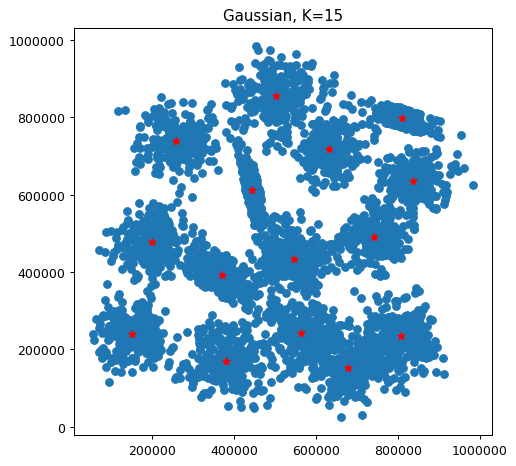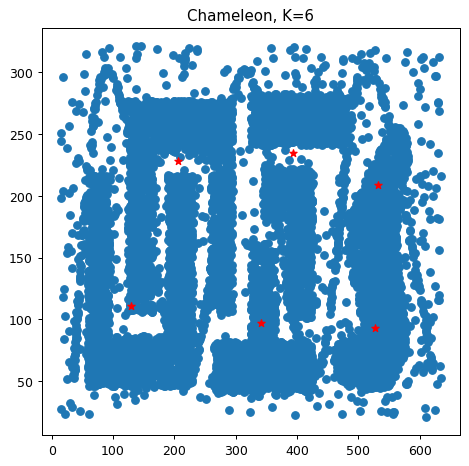### Exercise 1.7¶

We might be interested to the intermediate position of centroids, other than the final ones. We included this plotting functionality in the KMeans class defined above via the plot_clusters and plot_steps parameters of fit_predict. The plot is created in __plot_clusters. Take a close look at the c and cmap parameters. c accepts a list used to specify which points must have the same color. Points at indices with equal values in that list will have the same color. cmap instead lets you choose a color map among the ones predefined.

Let's test it with Gaussian clusters.

In :
np.random.seed(6)
k_gauss = KMeans(15)
_ = k_gauss.fit_predict(X_gauss, True, 3)

Early stopping at iteration 14!